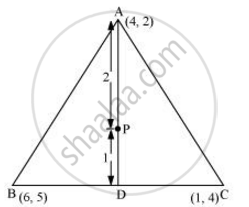# Let a (4, 2), B (6, 5) and C (1, 4) Be the Vertices δAbc. (I) the Median from a Meets Bc at D. Find the Coordinates of Point D. (Ii) Find the Coordinates of the Point P on Ad Such that Ap: Pd = 2:1 - Mathematics

Let A (4, 2), B (6, 5) and C (1, 4) be the vertices of ΔABC.

(i) The median from A meets BC at D. Find the coordinates of point D.

(ii) Find the coordinates of the point P on AD such that AP: PD = 2:1

(iii) Find the coordinates of point Q and R on medians BE and CF respectively such that BQ: QE = 2:1 and CR: RF = 2:1.

(iv) What do you observe?

(v) If A(x1, y1), B(x2, y2), and C(x3, y3) are the vertices of ΔABC, find the coordinates of the centroid of the triangle.

#### Solution(i) Median AD of the triangle will divide the side BC into two equal parts.

Therefore, D is the mid-point of side BC

Coordinate of D =  ((6+1)/2, (5+4)/2) = (7/2, 9/2)

(ii) Point P divides the side AD in a ratio 2:1.

Coordinate of P =((2xx7/2+1xx4)/(2+1), (2xx9/2+1xx2)/(2+1))= (11/3, 11/3)

(iii) Median BE of the triangle will divide the side AC into two equal parts.

Therefore, E is the mid-point of the side AC.

Coordinate of E = ((4+1)/2,(2+4)/2) = (5/2, 3)

Point Q divides the side BE in a ratio 2:1.

Coordinate of Q =((2xx5/2+1xx6)/(2+1), (2xx3+1xx5)/(2+1)) = (11/3,11/3)

Median CF of the triangle will divide the side AB in two equal parts. Therefore, F is the mid-point of side AB

Coordinate of F =((4+6)/2, (2+5)/2) = (5, 7/2)

Point R divides the side CF in a ratio 2:1.

Coordinate of R =((2xx5+1xx1)/(2+1),(2xx7/2+1xx4)/(2+1)) = (11/3, 11/3)

(iv) It can be observed that the coordinates of point P, Q, R are the same.

Therefore, all these are representing the same point on the plane i.e., the centroid of the triangle.

(v) Consider a triangle, ΔABC, having its vertices as A(x1, y1), B(x2, y2), and C(x3, y3).

The median AD of the triangle will divide the side BC into two equal parts. Therefore, D is the mid-point of side BC.

Coordinate of D =((x_2+x_3)/2,(y_2+y_3)/2)

Let the centroid of this triangle be O.

Point O divides the side AD in a ratio of 2:1.

Coordinate of O = ((2xx(x_2+x_3)/2+1xxx_1)/(2+1), (2xx(y_2+y_3)/2+1xxy_1)/(2+1))

= ((x_1+x_2+x_3)/3, (y_1+y_2+y_3)/3)

Concept: Area of a Triangle
Is there an error in this question or solution?
Chapter 6: Co-Ordinate Geometry - Exercise 6.3 [Page 31]

#### APPEARS IN

RD Sharma Class 10 Maths
Chapter 6 Co-Ordinate Geometry
Exercise 6.3 | Q 54 | Page 31
NCERT Class 10 Maths
Chapter 7 Coordinate Geometry
Exercise 7.4 | Q 7 | Page 171
Share